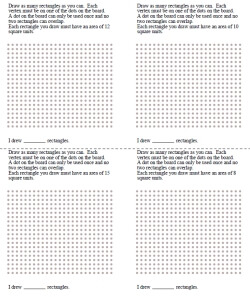edHelper.com Math Critical Thinking Geometry Drawing Shapes Challenge Lesson - Dot Game - Printable Activity Lesson Plan and Worksheets Drawing Shapes Challenge with Area of Shapes GivenWorksheets, Lessons, and Printables

Area: Given One Shape and Area - Draw as many as you canDraw as many rectangles as you can (area for each rectangle is given) Draw as many squares as you can (area for each square is given)

Drawing Shapes Challenge

Have a suggestion or would like to leave feedback?